•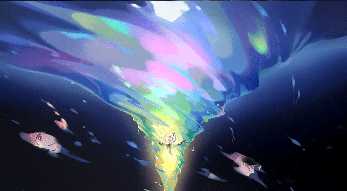深海火种

•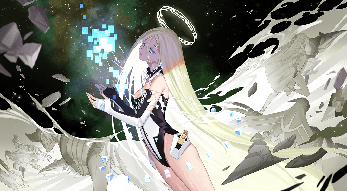星之原野

•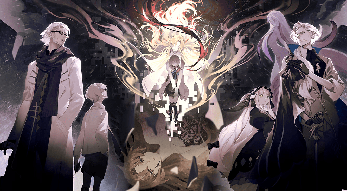神所留下的

•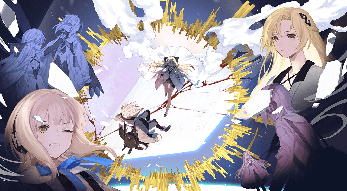导师与学生

•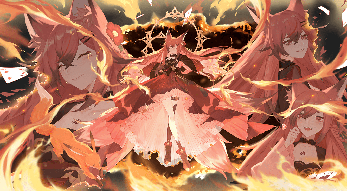终末的花火

•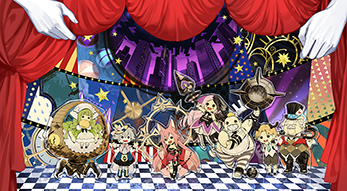奇异恩典

•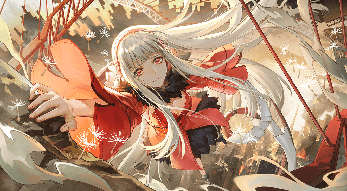期冀者的飘零

•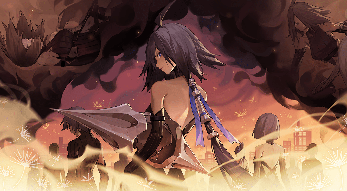飘零者的期冀

•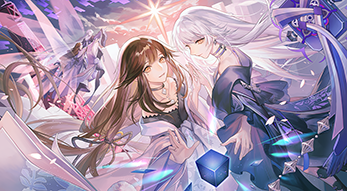门扉之外

•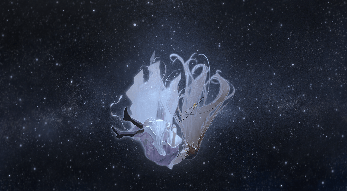微光

•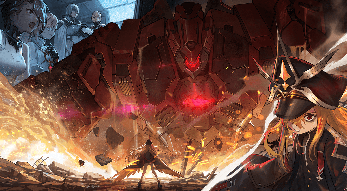废土机神

•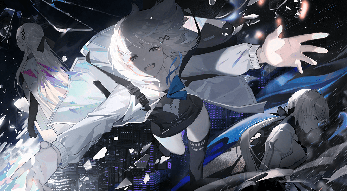相拥之时

•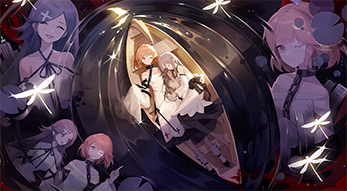虚妄之月

•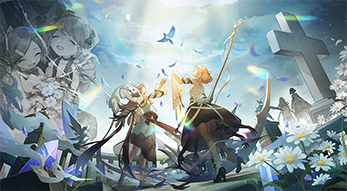琉璃之鸟

•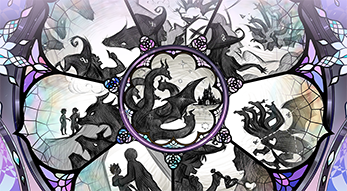怪物的心脏

•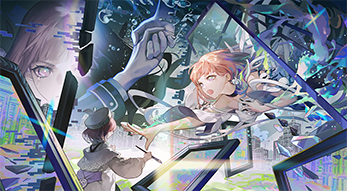幻彩之扉

•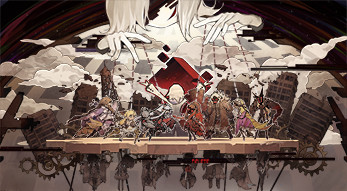箱庭风景

•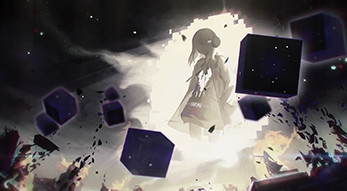终结

•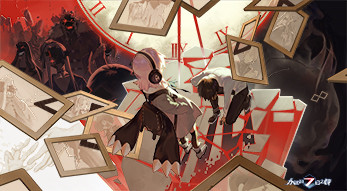牺牲的意义

•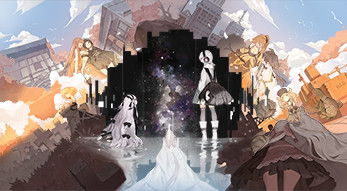深蓝之星

•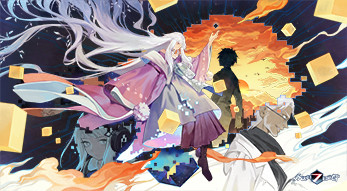黑暗中的身影

•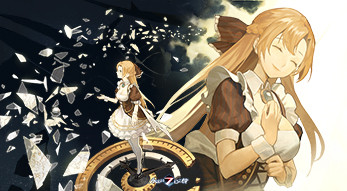两个人的旅途

•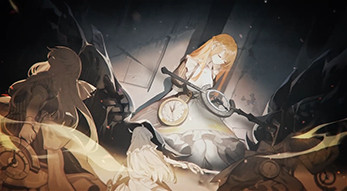永恒的终焉

•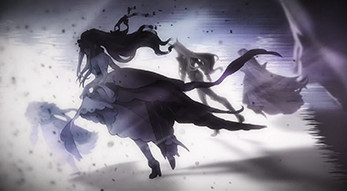被抛下的人

•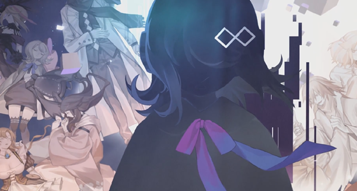神的棋盘

•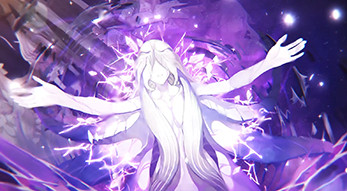零的故事

•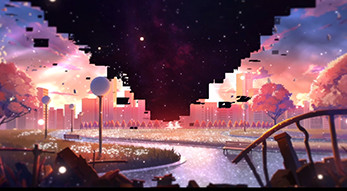两个人的城市

•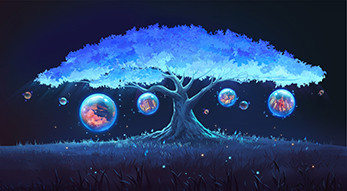记忆的种子

•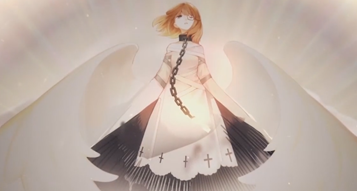纯白的救济

•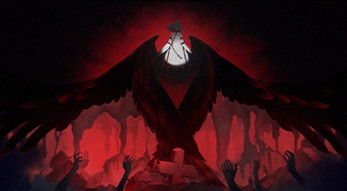漆黑的终音

•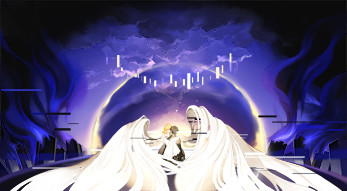折翼残响男

•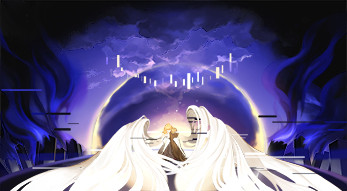折翼残响女

•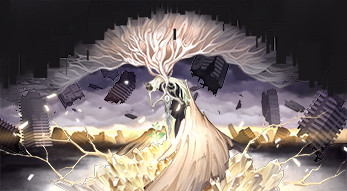庇护之树

•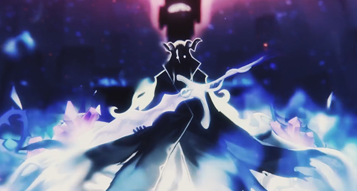神之嘲讽

•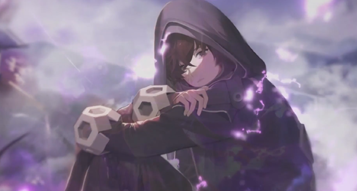黄昏的祈祷者

•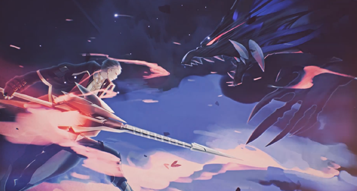黎明的见证者

•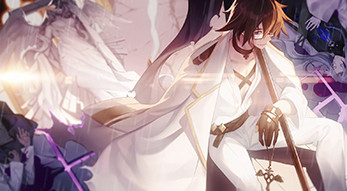守夜人

•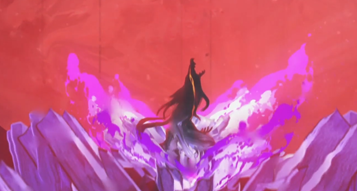子夜的毁灭者

•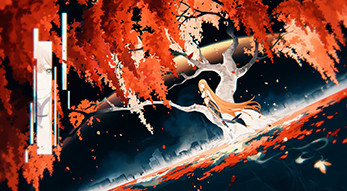没有你的世界

•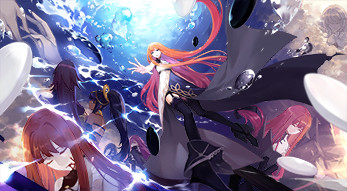紧紧相握的双手

•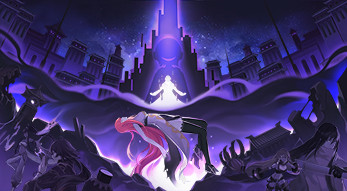觐见神明之人

•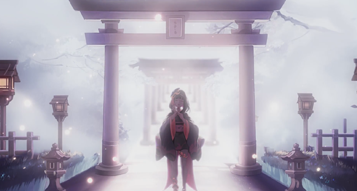轮回

•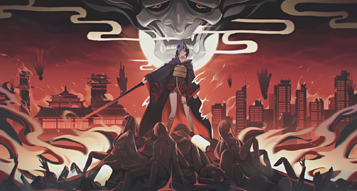业火

•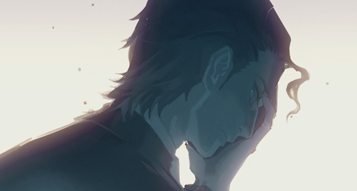白夜之笼

•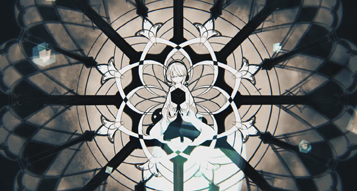神明坠落

•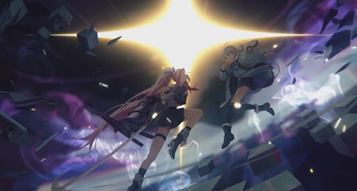神之一闪

•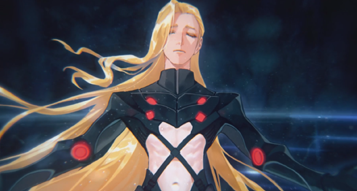破碎之日

•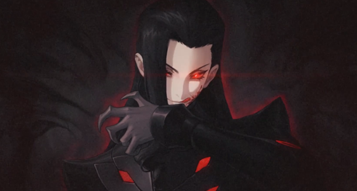来自地狱的天使

•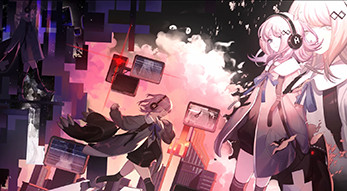未竟之愿

•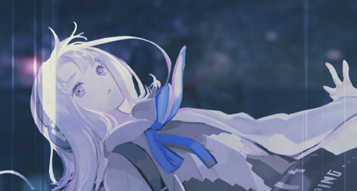告罪

•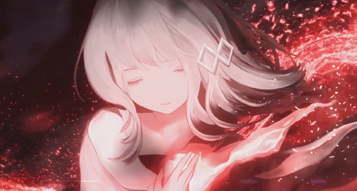心之空穴

•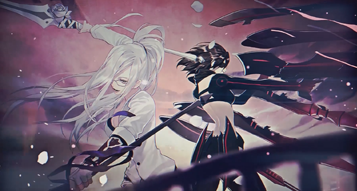与你并肩

•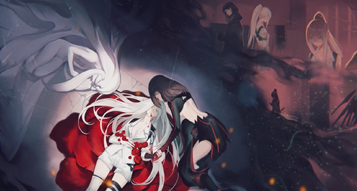希望已死

•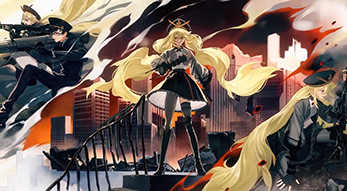永不磨灭的光芒

•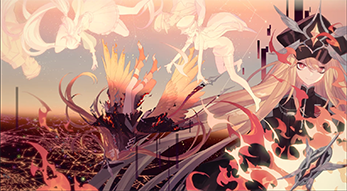星辰坠落之时

•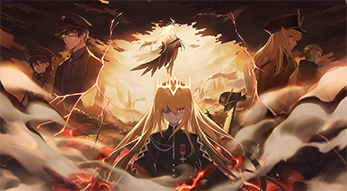最后的战役

•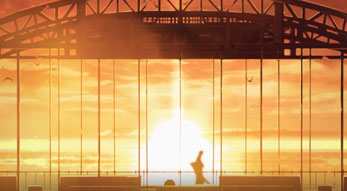鏖战

•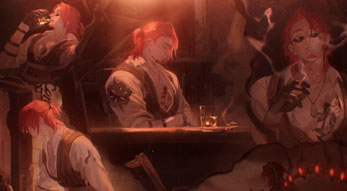最后一杯酒

•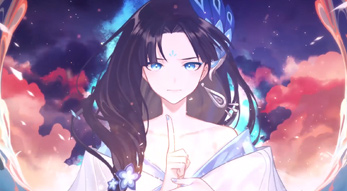复还

•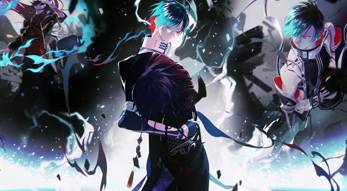无尽寂地

•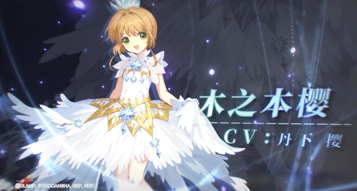小樱的7日冒险

•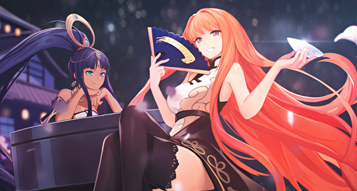东方书卷之始

•堕天使的挽歌

•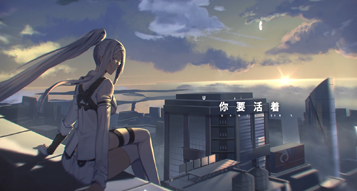黒匣白花

•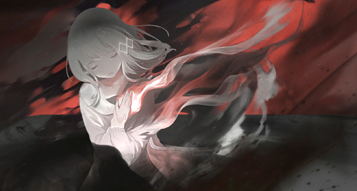深渊回声

•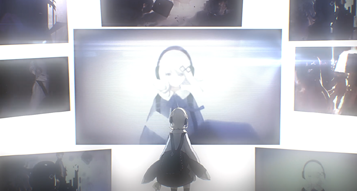箱庭之钥

•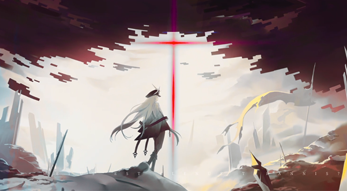破晓之翼

•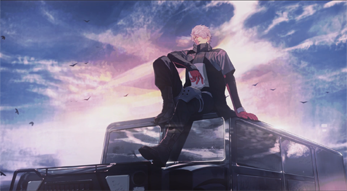赤贯的陨星

•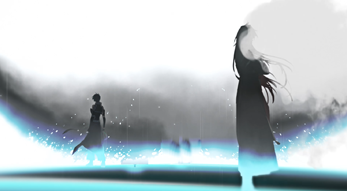终焉的命轮

•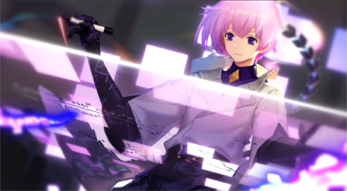永恒的信标

•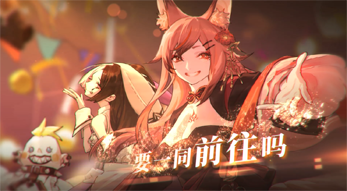致哀歌以欢笑

•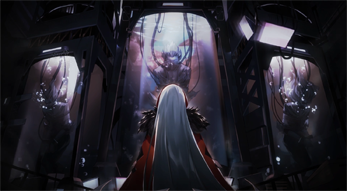逆光的勋章

•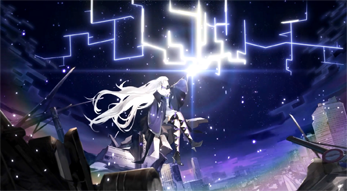浮世的镜影

•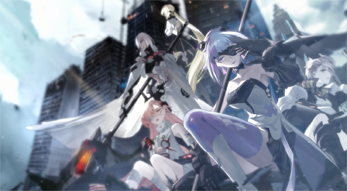风起之时

•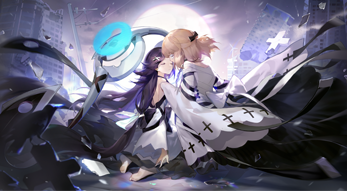碧空遥响

•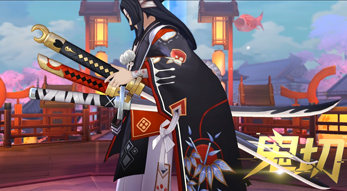式神7日都市之旅

•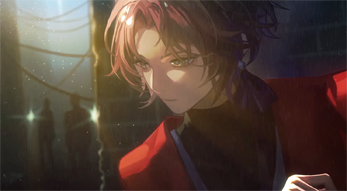乱序的夏弥尔

•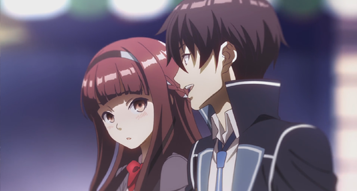主题曲：Mydays

•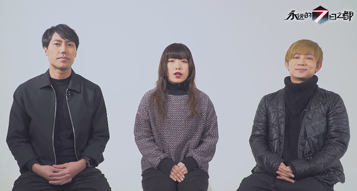MythRoid专访：《追想輪廻》创作心路

•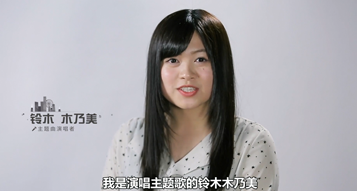铃木木乃美GARNiDELiA专访：《永远的7日之都》主题曲创作历程

•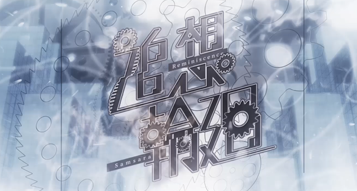追想輪廻

•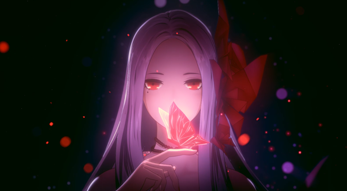无光的审理者

•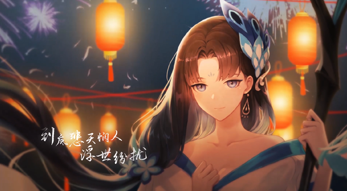如常

•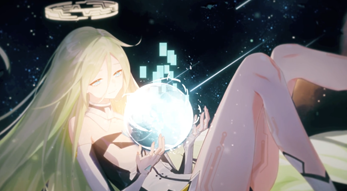依存之夜

•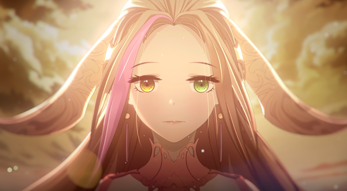无翼的月光鸟

•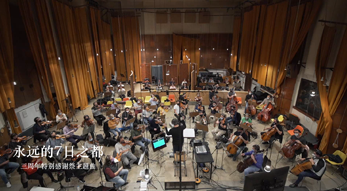7日之都大圆舞曲下载图片